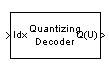# Quantizing Decoder

Decode quantization index according to codebook

## Library

Source Coding

•## Description

The Quantizing Decoder block converts quantization indices to the corresponding codebook values. The Quantization codebook parameter, a vector of length N, prescribes the possible output values. If the input is an integer k between 0 and N-1, then the output is the (k+1)st element of Quantization codebook.

The input must be a discrete-time signal. This block processes each vector element independently. For information about the data types each block port supports, see the Supported Data Type table on this page.

Note

The Quantizing Encoder block also uses a Quantization codebook parameter. The first output of that block corresponds to the input of Quantizing Decoder, while the second output of that block corresponds to the output of Quantizing Decoder.

## Parameters

Quantization codebook

A real vector that prescribes the output value corresponding to each nonnegative integer of the input.

Quantized output data type

Select the output data type.

## Supported Data Type

PortSupported Data Types
Idx
• Double-precision floating point

• Single-precision floating point

• 8-, 16-, and 32-bit signed integers

• 8-, 16-, and 32-bit unsigned integers

Q(U)
• Double-precision floating point

• Single-precision floating point

## Pair Block

Quantizing Encoder